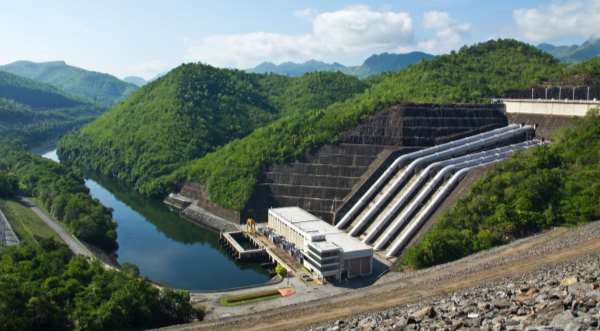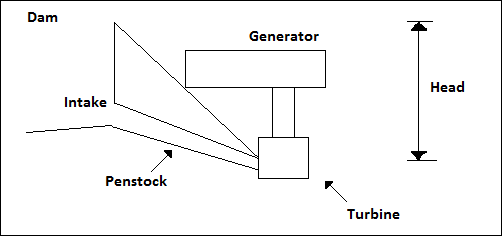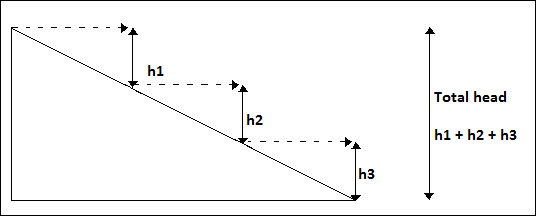# Hydroelectric Power - Introduction

Hydro Electric power (HEP) is a major renewable energy source used all over the world today to produce electricity. It utilizes the basic laws of Physics. Falling water under high pressure has high kinetic energy. In an HEP station, the falling water turns the turbines. Through magnetic induction, the generator converts the mechanical energy of the turbines to electricity.## Hydroelectric Power Station

It is the technique of using dam water falling from a height to turn the turbines of a generator. The mechanical energy is converted into electrical form and fed into the national grid system. The following diagram shows an outline of a hydroelectric power station.The location of a hydroelectric power station must be analyzed by an expert to determine the effective head for maximum efficiency. Hydraulic systems are also used to utilize the concept on slower and slow moving water streams.

One advantage of hydropower is that the water is available for other use after generation. A river with high water flow and head is a better source of hydropower.

Flow rate means the speed at which water passes a particular point in the river per second. The head refers to the vertical distance from the top of the slope to the power station.

A dam with a large drop is constructed to raise the potential energy of water. The intake is placed at the bottom where the pressure is highest. Water then flows by gravity through the penstock. At this level, kinetic energy is sufficient to turn the turbines.

## Power Estimation

The power in a dam can be estimated by two factors - flow of the water and the head.

• Flow means the volume passing through a given section of a river at a given time. Flow is given by m3/s.

• Head is the vertical distance the water falls through.

Theoretically, Power is directly proportional to the above mentioned factors, i.e.

P = Q*H*c

Where,

• P − power expected

• Q − the flow in m3/s

• H − Head in m

• c − constant (density* gravity)

Therefore, taking density of water as 1000gm-3 and gravity 1.9 −

P = 1000*1.9*Q*H

Energy is required to turn the turbines of a generator to cause an electromagnetic induction.

Pumped storage is a technique used to recycle water after it has passed through the turbines. In particular, pumped storage improves the overall dam’s efficiency.

A hydroelectric power station has three major components. They are −

• The first is the dam that creates the water head. Water falls from the base of the dam at high velocity and provides kinetic energy to turn the turbines.

• The second component is the reservoir. The water reservoir is the place behind the dam where water is stored. The water in the reservoir is located higher than the rest of the dam structure. The height of water in the reservoir decides how much potential energy the water possesses. The higher the height of water, the more its potential energy.

• The third component is the electric plant where electricity is produced and connected to the grid.

## Resource Assessment for Small Installation

Before installing a mini-hydro plant, it is important to identify nearby resource that can be harnessed. A good stream with a fairly constant flow (m3/s) is a resource worth exploiting.

A river with good flow can utilize the velocity of the water to turn the water wheel. Mountain or hill slopes are best suited for hydro-generation. As mentioned before, it is necessary to consider both head and flow of the river to determine the approximate power output.

Knowing the parameters, approximate power is determined as follows −

Head in feet * flow in gallons per meter / 10 = power in Watts

The head could also have the units of Pressure for an even river.

## Hose Tube Method

This technique is used in determining the head in a low stream for a submersed turbine.

The requirements for a hose tube method include a flexible piping (preferred garden horse), a funnel, and a measuring material. The stream should be shallow enough for one to wade through (check river depth before beginning). The procedure for installing a hose tube method is described below.

First, stretch the hose from the point where the stream starts to slope. Second, lift the end of the hose till water stops flowing through. Take the vertical distance and repeat the same for other sections till the preferred site is reached. The figure below illustrates the various heads in each section.